ESGS Logical Fallacies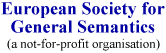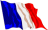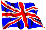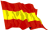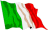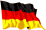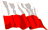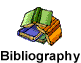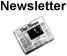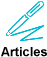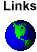Affirming the Consequent

 Definition

Also called Confusing A Sufficient With A Necessary Cause.

Any argument of the following form is invalid: If p1 then p2; p2; therefore p1.

 Explanation

This is mathematically incorrect. p1 is a sufficient cause of p2 and that means that if we have not-p2, we can conclude that we have not-p1 (the negation of "if p1 then p2"). But having p2 is not enough to conclude that we have p1.

 Examples

If I am in Calgary, then I am in Alberta. I am in Alberta, thus, I am in Calgary.
Of course, even though the premises are true, I might be in Edmonton, Alberta.

If the mill were polluting the river then we would see an increase in fish deaths. And fish deaths have increased. Thus, the mill is polluting the river.

 Counter-examples

None.